# Multiple Choice QuestionsAnonymous

### Question Description

1.

Given that the antiderivative of f(x) = e4x is F(x) =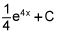, evaluate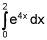. (4 points)

 e8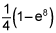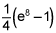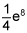2.

Evaluate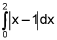. (4 points)

 0 1 2 None of these

3.

Given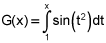, find G '(x). (4 points)

 2xsin(x2) 2xcos(x2) sin(x2) - sin(1) sin(x2)

4.

Find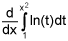. (4 points)

 2xln(x2)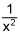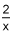ln(x2)

5.

Determine the interval on which f(x) = ln(x) is integrable. (4 points)

 (0, ∞) [0, ∞) (-∞, 0) U (0, ∞) All reals

6.
Which of the following sums does not equal the others? (4 points)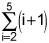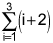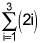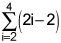7.

Write the summation to estimate the area under the curve y = 2x2 + 1 from x = 0 to x = 4 using 4 rectangles and left endpoints. (4 points)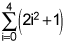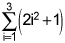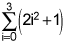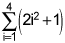School: UC BerkeleyPlease find all answers in attached DOC and PDF. Both files are exactly same content-wise. Please let me know if you have any doubt.Thanks

𝑒 8 −1
)
4

1. 𝑂�...

flag Report DMCAReviewAnonymous
Top quality work from this tutor! I’ll be back!Anonymous
Just what I needed… fantastic!Anonymous
Use Studypool every time I am stuck with an assignment I need guidance.Studypool4.7Trustpilot4.5Sitejabber4.4Brown University

1271 TutorsCalifornia Institute of Technology

2131 TutorsCarnegie Mellon University

982 TutorsColumbia University

1256 TutorsDartmouth University

2113 TutorsEmory University

2279 TutorsHarvard University

599 TutorsMassachusetts Institute of Technology

2319 TutorsNew York University

1645 TutorsNotre Dam University

1911 TutorsOklahoma University

2122 TutorsPennsylvania State University

932 TutorsPrinceton University

1211 TutorsStanford University

983 TutorsUniversity of California

1282 TutorsOxford University

123 TutorsYale University

2325 Tutors